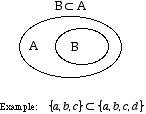index: click on a letter A B C D E F G H I J K L M N O P Q R S T U V W X Y Z A to Z index index: subject areas numbers & symbols sets, logic, proofs geometry algebra trigonometry advanced algebra & pre-calculus calculus advanced topics probability & statistics real world applications multimedia entrieswww.mathwords.com about mathwords website feedback

 Subset Set A is a subset of set B if all of the elements (if any) of set A are contained in set B. This is written A ⊂ B. Note: The empty set is a subset of every set.See also